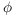# Group Maps Between Finite Cyclic Groups

Group mapsare determined by the image of: The image is an element whose order divides, and all such elements are the image of such a group map.

Theorem.

(a) Ifis a group map, then.

(b) Ifsatisfies, then there is a group mapsuch that.

Proof. (a) Supposeis a group map. Nowin, soThis shows that.

Since, I have.

Hence,.

(b) Let, and suppose. DefinebySince, I havefor some.

NowSince g sendsto 0, the Universal Property of the Quotient produces a (unique) group mapdefined byThen, andis the desired group map.Corollary. The number of group mapsis.

Proof. The number of elements of order d in a cyclic group is(wheredenotes the Euler-function). The divisor sum of the Euler-function is the identity:So the number of elements whose orders divideis, and the theorem shows that each such element gives rise to a group map.Example. (a) Enumerate the group maps.

(b) Show by direct computation thatgiven byis not a group map.

(a) Since, there are 6 such maps by the Corollary. They are determined by sendingto an element whose order divides 6.Thus, the possible group mapshaveFor example, the group mapIt is easy to determine the kernel and the image. The image is the unique subgroup ofof order 3, soBy the First Isomorphism Theorem, the kernel must have order. The unique subgroup ofof order 6 is(b) Consider the functiongiven by.ThenTherefore,, so f is not a group map.Contact information

Copyright 2016 by Bruce Ikenaga